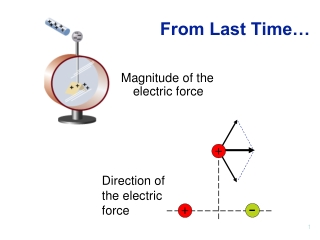DownloadDownload PresentationFrom Last Time…

# From Last Time…

Télécharger la présentation## From Last Time…

- - - - - - - - - - - - - - - - - - - - - - - - - - - E N D - - - - - - - - - - - - - - - - - - - - - - - - - - -
##### Presentation Transcript

1. + + From Last Time… Magnitude of the electric force Direction of the electric force

2. Induced dipoles (charge redistribution) charged rubber rod Bring negative charge close. Electrons on sphere move away from rod.

3. Exam 1 Tue. Sep. 29, 5:30-7 pm, 145 Birge Covers Chap. 21.5-7, 22, 23.1-4, 23.7, 24.1-5, 26 + lecture, lab, discussion, HW 8 1/2 x 11 handwritten note sheet (both sides) allowed • Study ideas: • Exam 1 Practice problems at Mastering Physics • Sample exams on website (blank & solutions) • Group/Quiz (blank & solutions). • Review lab question sheets. 3

4. Unusual dipoles:Electrogenic fish • Dipole + nearby conducting object Some fish generate charge separation - electric dipole. Dipole is induced in nearby (conducting) fish Small changes detected by fish.

5. The idea of electric fields • EM wave made up of oscillating electric and magnetic fields. • But what is an electric field? • Electric field is a way to describe the force on a charged particle due to other charges around it. • Force = charge  electric field • The direction of the force is the direction of the electric field.

6. + + + + Q2 + Q1 Electric field of a point charge Force on this charge… …due to this charge = Force/charge Units? N/C

7. + Calculating the electric field r = 10 cm Q1=1µC

8. Electric field • Electric field vector defined at every point in space. • Gives magnitude and direction of force on test particle e.g. wind velocity (speed and direction) in different parts of the country.

9. Superposition with Electric Fields • At any point P, the total electric field due to a group of source charges equals the vector sum of electric fields of all the charges • Find the electric field due to q1, E1 • Find the electric field due to q2, E2 • E = E1 + E2 • Remember, the fields add as vectors

10. Question Which vector best represents the electric field at the red dot? A B E C D - -

11. Electric Field Direction • a) q is positive, F is directed away from q • b) The direction of E is also away from the positive source charge • c) q is negative, F is directed toward q • d) E is also toward the negative source charge

12. Qp=1.6x10-19 C + r = 1x10-10 m Relationship Between F and E • Fe = qE • Valid for a test charge that does not disturb the source charge distribution • If q is positive, F and E are in the same direction Electric field 1Å away from proton E (to the right)

13. Quick Quiz Which is the direction of the electric field at dot? A. Left B. Right C. Up D. Down E. Zero Away from positive charge (right) y + - x Net E field is to right.

14. Quick Quiz In this electric dipole, what is the direction of the electric field at point A? Up Down C) Left D) Right E) Zero A x=-a x=+a +Q -Q

15. Electric field: summary • Electric field -> will be a force on a charged particle. • This force ( and electric field) can arise from electric charges (via Coulomb’s law) • But once electric field is known, don’t need to know the charges that produce it.

16. The electric dipole • Can all be approximated by electric dipole. • Two opposite charges magnitude qseparated by distance s Dipole moment Vector Points from - charge to + charge Has magnitude qs

17. For Since points from - charge to + charge on y-axis of dipole only Calculating dipole electric field y On the y-axis q s x -q

18. A B Question: electric dipole A and B are the same (large) distance from dipole. How do the magnitude of the electric fields at A and B compare?

19. Pictorial representation of E: Electric Field Lines

20. Electric field lines • Local electric field tangent to field line • Density of lines proportional to electric field strength • Fields lines can only start on + charge • Can only end on - charge. • Electric field lines can never cross

21. + - Electric field of a dipole

22. Electric field of two + charges

23. A B Question How are the charges A and B related? A+, B-, A-, B+, A+, B-, A-, B+, A+, B-,